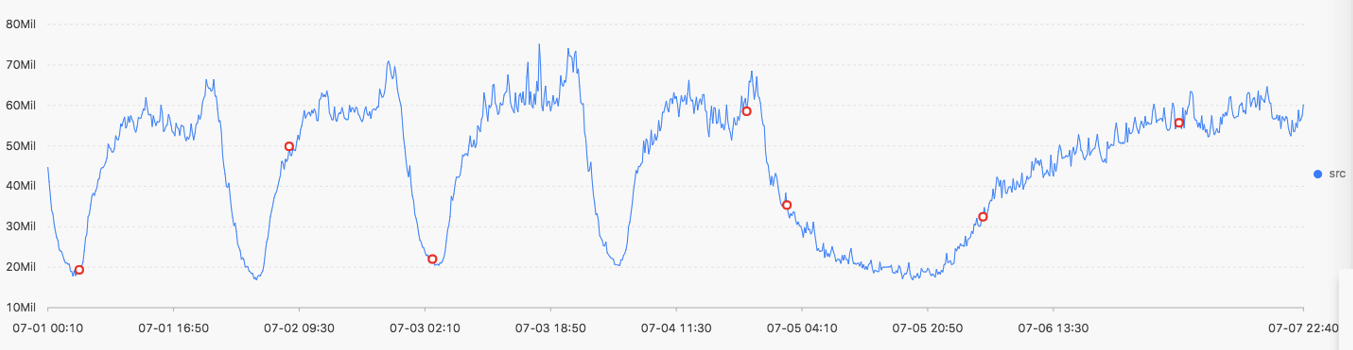This topic describes the change point detection functions that you can use to detect change points in time series data.

The change point detection functions can detect the following change points:
• Statistical feature changes within a specified period of time
• Anomalies in time series data

## Function list

Function Description
ts_cp_detect Detects intervals with different statistical features from time series data. The interval endpoints are change points.
ts_breakout_detect Detects the points in time when statistics steeply increase or decrease from time series data.

## ts_cp_detect

Function format:
• If you cannot specify a suitable time window length, you can use the following function. The default window length used in the function for detection is 10.
``select ts_cp_detect(x, y, samplePeriod)``
• To debug the effect specific to your business environment, you can specify the minSize parameter in the following function.
``select ts_cp_detect(x, y, minSize) ``
The following table lists the parameters of the function format.
Parameter Description Value
x The time sequence. Points in time are sorted in ascending order along the horizontal axis. Each point in time is a Unix timestamp. Unit: seconds.
y The sequence of numeric data corresponding to each specified point in time. N/A.
minSize The minimum length of time series data within a consecutive interval. The minimum length is 3. The maximum length cannot exceed one tenth of the length of specified time series data.
Example:
• The query statement is as follows:
``* | select ts_cp_detect(stamp, value, 3) from (select __time__ - __time__ % 10 as stamp, avg(v) as value from log GROUP BY stamp order by stamp) ``
• Output resultThe following table lists the display items.
Display item Description
Horizontal axis unixtime The timestamp of the point in time the data is recorded. Unit: seconds. Example: 1537071480.
Vertical axis src The raw data. Example: 1956092.7647745228.
prob The probability that a point in time is a change point. Valid values: [0, 1].

## ts_breakout_detect

Function format:
``select ts_breakout_detect(x, y, winSize) ``
The following table lists the parameters of the function format.
Parameter Description Value
x The time sequence. Points in time are sorted in ascending order along the horizontal axis. Each point in time is a Unix timestamp. Unit: seconds.
y The sequence of numeric data corresponding to each specified point in time. N/A.
winSize The minimum length of time series data within a consecutive interval. The minimum length is 3. The maximum length cannot exceed one tenth of the length of specified time series data.
Example:
• The query statement is as follows:
``* | select ts_breakout_detect(stamp, value, 3) from (select __time__ - __time__ % 10 as stamp, avg(v) as value from log GROUP BY stamp order by stamp) ``
• Output resultThe following table lists the display items.
Display item Description
Horizontal axis unixtime The timestamp of the point in time the data is recorded. Unit: seconds. Example: 1537071480.
Vertical axis src The raw data. Example: 1956092.7647745228.
prob The probability that a point in time is a change point. Valid values: [0, 1].